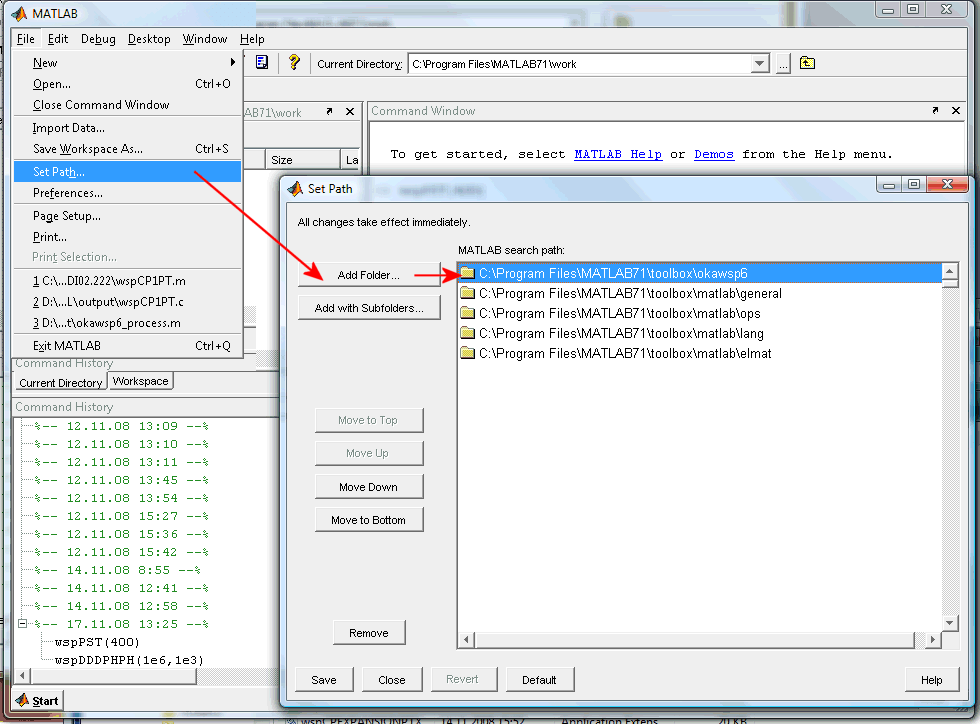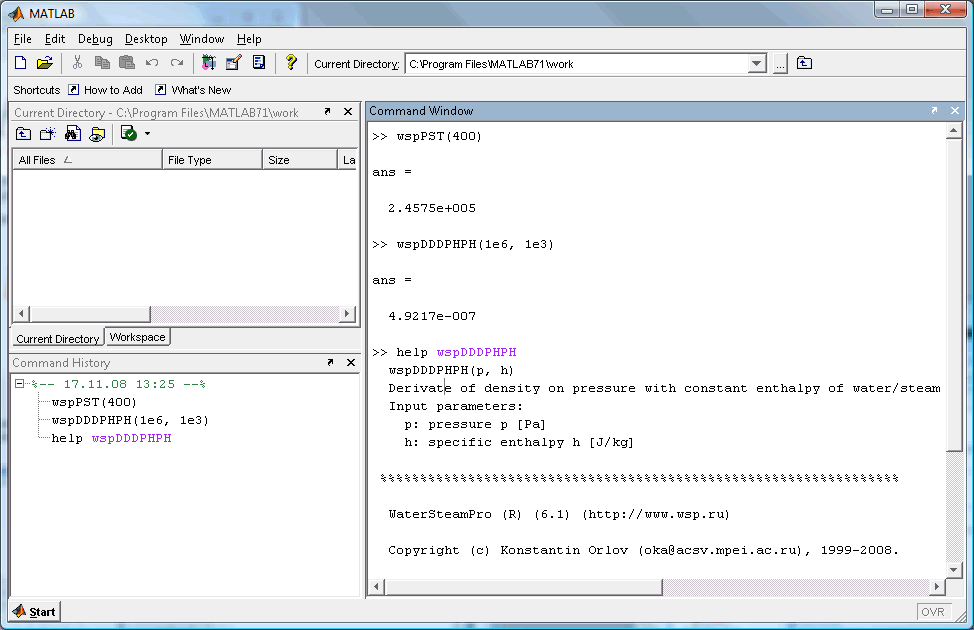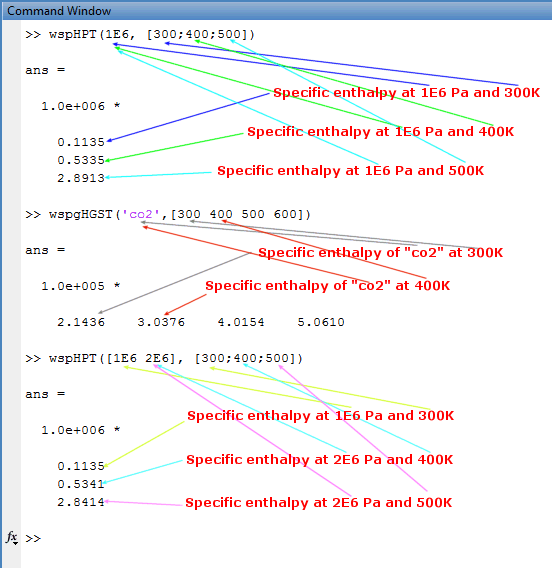# WaterSteamPro usage in MATLAB

There are several steps required in order to use WaterSteamPro in MATLAB.

2. Download archive with some files for using WaterSteamPro in MATLAB from WaterSteamPro WWW-site: http://www.wsp.ru (this step can be done).
3. Extract files from archive to any empty folder in your computer. It can be subfolder in MATLAB installation path:
4.5. Run MATLAB.
6. In MATLAB please go to "File" menu and select menu item "Set Path...". Press "Add Folder..." button and select the folder where the files are extracted (see step #3).
7.8. And you can use WaterSteamPro functions in MATLAB:
9.Note that the WaterSteamPro files for MATLAB are specially designed to allow use arguments with different dimensions and different count of array elements.

See the next image.You can see the example of call to WaterSteamPro functions.

1. The first one is the call to WaterSteamPro function "wspHPT(p, t)" which return specific enthalpy of water/steam for given pressure p and temperature t. And you can see that number of elements in arguments arrays for pressure (1E6 - one element) and temperature (300;400;500 - three elements) are not the same.

How this sitiation is processed?

When you call to WaterSteamPro function from MATLAB for first determined maximum number of elements in input arrays of arguments. Then the same number of output are created.

Later processed the call to WaterSteamPro function in "for" cycle for all elements in inputs.

But when the number of elements for one argument is not enough than the latest element is used.

In first sample pressure array have only one element (equal to 1E6) so this value used to calculate specific enthalpy for all temperatures (300K, 400K, 500K).

2. In second example you can see practically the same situation, but for function "wspgHGST(gas, t)" which calculates specific enthalpy of gas (in this case "co2") in ideal case for given temperature.

Number of elements in argument "gas" is 1. Number of elements in argument "t" is 4. So specific enthalpy calculates for one gas "co2" at 4 different temperatures (300K, 400K, 500K, 600K)

3. In third example you can see another type of situations. It is used the same function "wspHPT(p, t)" as in first sample.

But number of elements in p argument is 2 and in t argument is 3. How it is processed?

You can see that first element in output is calculated for first element in argument p and first element in argument t. The second one - is calculated for first element in argument p and first element in argument t

But the third element in output haven't third element in argument p (but have it in argument t). And the latest value from argument p is used (the second element in argument p)

MATLAB is registered trademark of Mathworks, Inc.